# Intermediate Algebra Tutorial 38

Intermediate Algebra
Tutorial 38: Rational Exponents

WTAMU > Virtual Math Lab > Intermediate AlgebraLearning Objectives

After completing this tutorial, you should be able to:
1. Rewrite a rational exponent in radical notation.
2. Simplify an expression that contains a rational exponent.
3. Use rational exponents to simplify a radical expression.Introduction

In this tutorial we are going to combine two ideas that have been discussed in earlier tutorials: exponents and radicals.  We will look at how to rewrite, simplify and evaluate these expressions that contain rational exponents.   What it boils down to is if you have a denominator in your exponent, it is your index or root number.  So, if you need to, review radicals covered in Tutorial 37: Radicals.  Also, since we are working with fractional exponents and they follow the exact same rules as integer exponents, you will need to be familiar with adding, subtracting, and multiplying them. If fractions get you down you may want to go to Beginning Algebra Tutorial 3: Fractions.  To review exponents, you can go to Tutorial 23: Exponents and Scientific Notation Part I and Tutorial 24: Exponents and Scientific Notation Part II.  Let's move onto rational exponents and roots.Tutorial

Rational Exponents and Roots

If x is positive, p and q are integers and q is positive,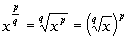In other words, when you have a rational exponent, the denominator of that exponent is your index or root number and the numerator of the exponent is the exponential part.

I have found it easier to think of it in two parts.  Find the root part first and then take it to the exponential part if possible.  It makes the numbers a lot easier to work with.

Radical exponents follow the exact same exponent rules as discussed in Tutorial 23: Exponents and Scientific Notation, Part I and Tutorial 24: Exponents and Scientific Notation, Part IIIn those two tutorials we only dealt with integers, but you can extend those rules to rational exponents.

Here is a quick review of those exponential rules:

Review of Exponential Rules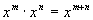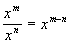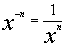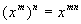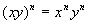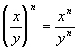Example 1: Use radical notation to write the expression and simplify.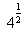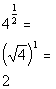*Rewrite exponent 1/2 as a square root

We are looking for the square root of 4 raised to the 1 power, which is the same as just saying the square root of 4.

If your exponent's numerator is 1, you are basically just looking for the root (the denominator's exponent).

Our answer is 2 since the square root of 4 is 2.Example 2: Use radical notation to write the expression and simplify.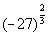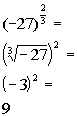In this problem we are looking for the cube root of -27 squared.  Again, I think it is easier to do the root part first if possible.  The numbers will be easier to work with.

The cube root of -27 is -3 and (-3) squared is 9.Example 3: Use radical notation to write the expression and simplify.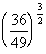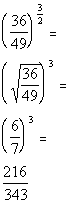In this problem we are looking for the square root of 36/49 cubed.  Again, I think it is easier to do the root part first if possible.  The numbers will be easier to work with.

The square root of 36/49 is 6/7 and 6/7 cubed is 216/343.Example 4: Write with a positive exponent and simplify.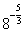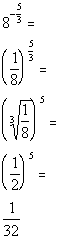In this problem we are looking for the cube root of 1/8 raised to the fifth power.  Again, I think it is easier to do the root part first if possible.  The numbers will be easier to work with.

The cube root of 1/8 is 1/2 and 1/2 raised to the fifth power is 1/32.Example 5: Simplify the expression.  Write with positive exponents only.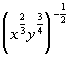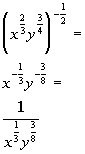Example 6: Simplify the expression. Write with positive exponents only.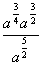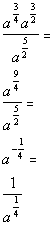Example 7: Multiply.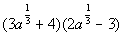Since this is a binomial times a binomial, we can use the FOIL method as discussed in Tutorial 26: Multiplying Polynomials.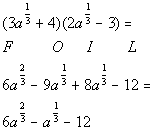Example 8:  Factor the common factor from the given expression.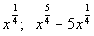Basically, we are factoring out a GCF as discussed in Tutorial 27: The GCF and Factoring by Grouping.  Remember when you factor out the GCF, you our doing the reverse of the distributive property.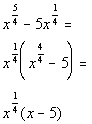*Factor out x to the 1/4Example 9:  Use rational exponents to simplify the radical.  Assume that the variable represents a positive number.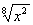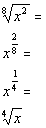*Simplify exponent
*Rewrite exponent 1/4 as a fourth rootPractice Problems

These are practice problems to help bring you to the next level.  It will allow you to check and see if you have an understanding of these types of problems. Math works just like anything else, if you want to get good at it, then you need to practice it.  Even the best athletes and musicians had help along the way and lots of practice, practice, practice, to get good at their sport or instrument.  In fact there is no such thing as too much practice.

To get the most out of these, you should work the problem out on your own and then check your answer by clicking on the link for the answer/discussion for that  problem.  At the link you will find the answer as well as any steps that went into finding that answer.Practice Problems 1a - 1c: Use radical notation to write the expression and simplify.

1a.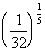1b.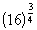1c.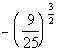Practice Problem 2a: Write with a positive exponent and simplify.

2a.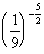Practice Problems 3a - 3b: Simplify the expression, write with positive exponents only.

3a.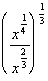3b.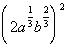Practice Problem 4a: Multiply.

4a.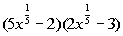Practice Problem 5a: Factor the common factor from the given expression.

5a.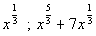Practice Problem 6a: Use rational exponents to simplify the radical.  Assume that the variable represents a positive number.

6a.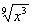Need Extra Help on these Topics?

Go to Get Help Outside the Classroom found in Tutorial 1: How to Succeed in a Math Class for some more suggestions.Last revised on July 19, 2011 by Kim Seward.